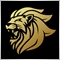# Fractal indicator47

Hello Guys, this is the list of Fractals free indicator:

```//+------------------------------------------------------------------+
//|                                                     Fractals.mq4 |
//|                                        http://www.metaquotes.net |
//+------------------------------------------------------------------+

#property indicator_chart_window
#property indicator_buffers 2
#property indicator_color1 Red
#property indicator_color2 YellowGreen
//---- input parameters

//---- buffers
double ExtUpFractalsBuffer[];
double ExtDownFractalsBuffer[];
datetime TempoSalvato=NULL;
datetime TS=NULL;
//+------------------------------------------------------------------+
//| Custom indicator initialization function                         |
//+------------------------------------------------------------------+
int init()
{
//---- indicator buffers mapping
SetIndexBuffer(0,ExtUpFractalsBuffer);
SetIndexBuffer(1,ExtDownFractalsBuffer);
//---- drawing settings
SetIndexStyle(0,DRAW_ARROW);
SetIndexArrow(0,119);
SetIndexStyle(1,DRAW_ARROW);
SetIndexArrow(1,119);
//----
SetIndexEmptyValue(0,0.0);
SetIndexEmptyValue(1,0.0);
//---- name for DataWindow
SetIndexLabel(0,"Fractal Up");
SetIndexLabel(1,"Fractal Down");
//---- initialization done
return(0);
}
//+------------------------------------------------------------------+
//| Custor indicator deinitialization function                       |
//+------------------------------------------------------------------+
int deinit()
{

//----
return(0);
}
//+------------------------------------------------------------------+
//| Custom indicator iteration function                              |
//+------------------------------------------------------------------+
int start()
{
int    i,nCountedBars;
bool   bFound;
double dCurrent;
nCountedBars=IndicatorCounted();
//---- last counted bar will be recounted
if(nCountedBars<=2)
i=Bars-nCountedBars-3;
if(nCountedBars>2)
{
nCountedBars--;
i=Bars-nCountedBars-1;
}
//----Up and Down Fractals
while(i>=2)
{
//----Fractals up

if (TempoSalvato!=Time)
{
TempoSalvato=Time;
// Alert("fractals up"+Symbol()+" a TF "+IntegerToString(Period(),0,0));
}
bFound=false;
dCurrent=High[i];
if(dCurrent>High[i+1] && dCurrent>High[i+2] && dCurrent>High[i-1] && dCurrent>High[i-2])
{
bFound=true;
ExtUpFractalsBuffer[i]=dCurrent;
}
//----6 bars Fractal
if(!bFound && (Bars-i-1)>=3)
{
if(dCurrent==High[i+1] && dCurrent>High[i+2] && dCurrent>High[i+3] &&
dCurrent>High[i-1] && dCurrent>High[i-2])
{
bFound=true;
ExtUpFractalsBuffer[i]=dCurrent;
}
}
//----7 bars Fractal
if(!bFound && (Bars-i-1)>=4)
{
if(dCurrent>=High[i+1] && dCurrent==High[i+2] && dCurrent>High[i+3] && dCurrent>High[i+4] &&
dCurrent>High[i-1] && dCurrent>High[i-2])
{
bFound=true;
ExtUpFractalsBuffer[i]=dCurrent;
}
}
//----8 bars Fractal
if(!bFound && (Bars-i-1)>=5)
{
if(dCurrent>=High[i+1] && dCurrent==High[i+2] && dCurrent==High[i+3] && dCurrent>High[i+4] && dCurrent>High[i+5] &&
dCurrent>High[i-1] && dCurrent>High[i-2])
{
bFound=true;
ExtUpFractalsBuffer[i]=dCurrent;
}
}
//----9 bars Fractal
if(!bFound && (Bars-i-1)>=6)
{
if(dCurrent>=High[i+1] && dCurrent==High[i+2] && dCurrent>=High[i+3] && dCurrent==High[i+4] && dCurrent>High[i+5] &&
dCurrent>High[i+6] && dCurrent>High[i-1] && dCurrent>High[i-2])
{
bFound=true;
ExtUpFractalsBuffer[i]=dCurrent;
}
}
//----Fractals down
if (TempoSalvato!=Time)
{
TempoSalvato=Time;
}
bFound=false;
dCurrent=Low[i];
if(dCurrent<Low[i+1] && dCurrent<Low[i+2] && dCurrent<Low[i-1] && dCurrent<Low[i-2])
{
bFound=true;
ExtDownFractalsBuffer[i]=dCurrent;
}
//----6 bars Fractal
if(!bFound && (Bars-i-1)>=3)
{
if(dCurrent==Low[i+1] && dCurrent<Low[i+2] && dCurrent<Low[i+3] &&
dCurrent<Low[i-1] && dCurrent<Low[i-2])
{
bFound=true;
ExtDownFractalsBuffer[i]=dCurrent;
}
}
//----7 bars Fractal
if(!bFound && (Bars-i-1)>=4)
{
if(dCurrent<=Low[i+1] && dCurrent==Low[i+2] && dCurrent<Low[i+3] && dCurrent<Low[i+4] &&
dCurrent<Low[i-1] && dCurrent<Low[i-2])
{
bFound=true;
ExtDownFractalsBuffer[i]=dCurrent;
}
}
//----8 bars Fractal
if(!bFound && (Bars-i-1)>=5)
{
if(dCurrent<=Low[i+1] && dCurrent==Low[i+2] && dCurrent==Low[i+3] && dCurrent<Low[i+4] && dCurrent<Low[i+5] &&
dCurrent<Low[i-1] && dCurrent<Low[i-2])
{
bFound=true;
ExtDownFractalsBuffer[i]=dCurrent;
}
}
//----9 bars Fractal
if(!bFound && (Bars-i-1)>=6)
{
if(dCurrent<=Low[i+1] && dCurrent==Low[i+2] && dCurrent<=Low[i+3] && dCurrent==Low[i+4] && dCurrent<Low[i+5] &&
dCurrent<Low[i+6] && dCurrent<Low[i-1] && dCurrent<Low[i-2])
{
bFound=true;
ExtDownFractalsBuffer[i]=dCurrent;
}
}
i--;
}

if (TS!=Time)
{
TS=Time;
}

//----
return(0);
}
//+------------------------------------------------------------------+```

It has been modified so that every time a Fractal up appears, it should appear the alert "Alert (" A fractal UP was generated on "+ Symbol () +" TF: "+ Period ());" and every time a down alert appears, compares Alert ("A down Fractal was created on" + Symbol () + "TF:" + Period ()); "but it does not always compare can someone help me solve the problem?Moderator
12384

`dCurrent=High[i];`

When dCurrent ==0 then

```ExtUpFractalsBuffer!=0
And/or
ExtDownFractalsBuffer!=0

==0
```

So you add another alert for when those buffers ==0 to see when that happens and there you will hopefully see what and why it happens... don't forger to also print the value of i.19975

```if (ExtUpFractalsBuffer!=0 && AlertNonInviato){Alert("E' stato generato un frattale UP su "+Symbol()+ "TF:" + Period()); AlertNonInviato=False;}
```
There will never be a fractal on the current bar, only bar 247

whroeder1:
There will never be a fractal on the current bar, only bar 2

So this should be OK?

``` if (ExtUpFractalsBuffer!=0 && AlertNonInviato){Alert("E' stato generato un frattale UP su "+Symbol()); AlertNonInviato=False;}
```47

texcs81:

So this should be OK?

its ok!

tanks87

whroeder1:
There will never be a fractal on the current bar, only bar 2
texcs81:

Hello Guys, this is the list of Fractals free indicator:

It has been modified so that every time a Fractal up appears, it should appear the alert "Alert (" A fractal UP was generated on "+ Symbol () +" TF: "+ Period ());" and every time a down alert appears, compares Alert ("A down Fractal was created on" + Symbol () + "TF:" + Period ()); "but it does not always compare can someone help me solve the problem?

hello, im newbie

fractal buffer appears after close candle 1,

so, how to set fractal buffer after close candle 2. I tried but i dont find the answer.